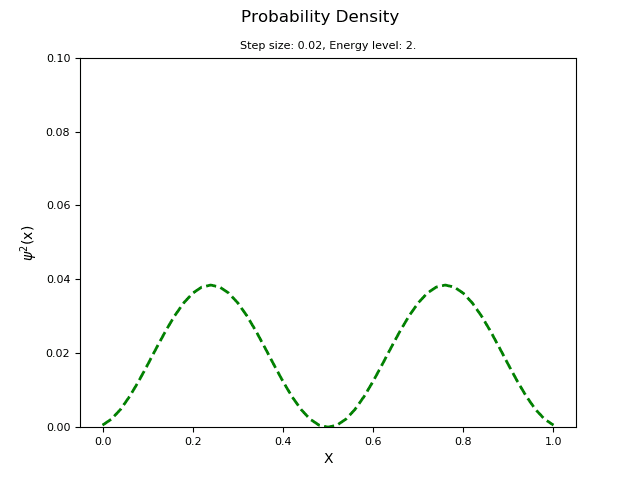# (Baby QM) Analytic Solution to the Infinite Square Well Problem

• I
Hi,

I think I'm having a bit of a brain fart...I'm messing with this numerical code trying to understand the 1-D time-independent Schrodinger's equation infinite square well problem (V(x) infinite at the boundaries, 0 everywhere else). If normalized Phi squared is the probability of finding the particle in that location, what the heck is the analytical solution I should plot against it to see how close it is? The normalized wavefunction given by Hyperphysics is much too large. Any and all suggestions appreciated.nrqed
Homework Helper
Gold Member
Hi,

I think I'm having a bit of a brain fart...I'm messing with this numerical code trying to understand the 1-D time-independent Schrodinger's equation infinite square well problem (V(x) infinite at the boundaries, 0 everywhere else). If normalized Phi squared is the probability of finding the particle in that location, what the heck is the analytical solution I should plot against it to see how close it is? The normalized wavefunction given by Hyperphysics is much too large. Any and all suggestions appreciated.
View attachment 260814
What do you mean their wavefunction is too large? What specific wavefunction were you looking at?

hilbert2